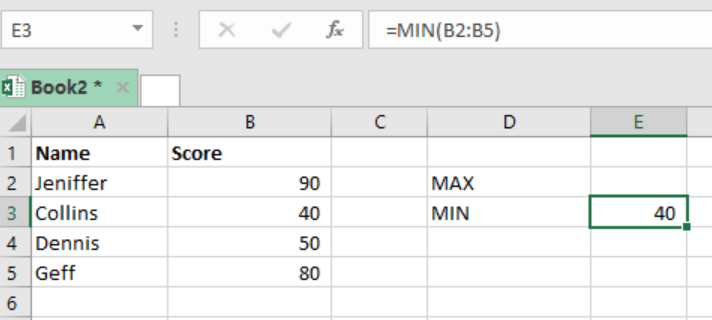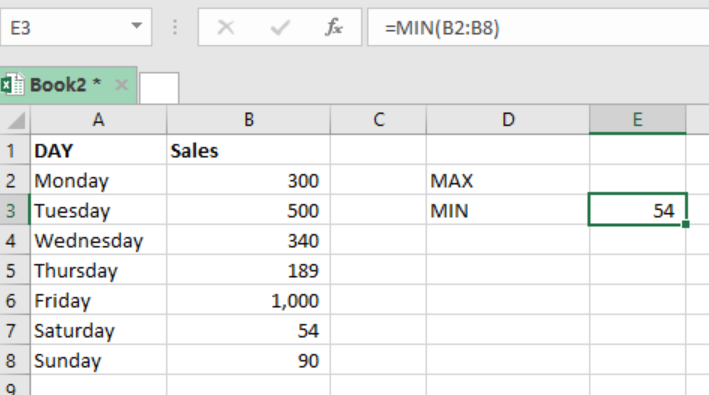Get instant live expert help with Excel or Google Sheets“My Excelchat expert helped me in less than 20 minutes, saving me what would have been 5 hours of work!”

#### Post your problem and you’ll get Expert help in seconds.

Your message must be at least 40 characters
Our professional Expert are available now. Your privacy is guaranteed.

# Cell Contains All of Many Things

We can use a formula that deploys the SEARCH function, with the help of ISNUMBER, SUMPRODUCT and COUNTA function to test if a cell contains all of many things in a list. For you to understand how this is done, here is a post that provides a clear guide about it.Figure 1: Testing if cell contains all of many things

## Syntax of the formula

`=SUMPRODUCT (--ISNUMBER (SEARCH (things, A1))) =COUNTA (things)`

Where;

• `=ISNUMBER (SEARCH (things, A1)` – is responsible for searching a single substring in a cell. If the cell contains a substring, the result is TRUE, if it doesn’t, then it is a FALSE.
• Double negative (-) – converts TRUE into 1 and FALSE to 0.
• SUMPRODUCT- sums the array and gives us total count. If total equals number of products inserted in “things”, result will be TRUE.
• COUNTA- identifies non-blank cells in “things”.

## How the formula works

• This formula plays a fundamental role when it comes to testing whether a cell contains all of many things.
• The formula uses SEARCH function with the help of ISNUMBER, SUMPRODUCT as well as the COUNTA functions.
• The formula counts all matches present in the list just ones.
• It then compares the count of the products we want that are present in the list.
• If the two are similar, the all the products are listed in the cell and the formula will return a TRUE result.
• If the two are not similar, the formula will return a FALSE result.

## ExampleFigure 2: testing if cell contains many of all things

• In our example above, our text string range is B2:B5
• We want to find out if all the cells contain all the words in range D2:D4
• We use the formula below to get the results as shown;

`=SUMPRODUCT(--ISNUMBER(SEARCH(D2:D5,C3)))=COUNTA(D2:D5)`

## Instant Connection to an Expert through our Excelchat Service

Most of the time, the problem you will need to solve will be more complex than a simple application of a formula or function. If you want to save hours of research and frustration, try our live Excelchat service! Our Excel Experts are available 24/7 to answer any Excel question you may have. We guarantee a connection within 30 seconds and a customized solution within 20 minutes.

### Did this post not answer your question? Get a solution from connecting with the expert.Another blog reader asked this question today on Excelchat:## Subscribe to Excelchat.coAnother blog reader asked this question today on Excelchat: